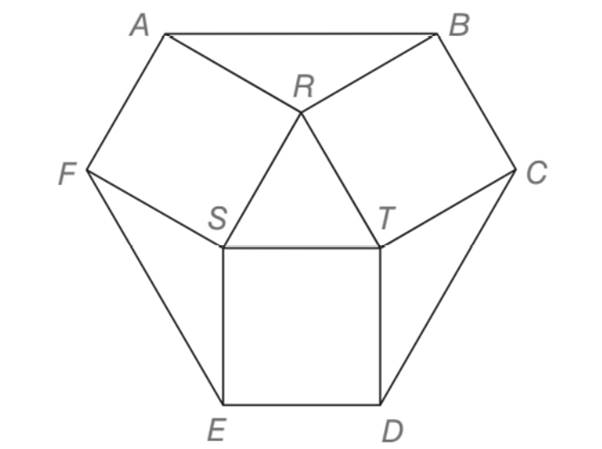Chapter 5.5, Problem 39EElementary Geometry For College St...

7th Edition
Alexander + 2 others
ISBN: 9781337614085

Solutions

Chapter
SectionElementary Geometry For College St...

7th Edition
Alexander + 2 others
ISBN: 9781337614085
Textbook Problem

For equilateral ∆ R S T , R T = 6 . Squares and triangles on the sides form hexagon A B C D E F . Find the perimeter of A B C D E F .To determine

To find:

The perimeter of the hexagon ABCDEF formed by squares and triangles on sides with an equilateral triangle RST, RT=6.

Explanation

Definition:

The perimeter of any figure is given by the sum of the total length of its boundary

Calculation:

Given,

RST is an equilateral triangle with RT=6

STDE, TRBC and RSFR are squares

RAB, TCD and SEF are triangles.

Since, RST is an equilateral triangle all the three sides of the triangle are congruent.

RS=ST=RT=6 units.

As such each of the squares STDE, TRBC and RSFR are formed with one of ifs sides in common with the equilateral triangle each side of all the three squares measures 6 units.

Further, two of the adjacent sides of the triangle RAB, TCD and SEF are formed by the sides of the square which in turn makes the triangle as an isosceles triangle with equal sides measure 6 units.

The interior angles formed at the vertex R, S and T of the triangle RAB, TCD and SEF is give by

=180°- the interior angle of the equilateral

=180°-60°

=120°

Now, consider one of the isosceles RAB and draw RL¯AB¯, which bisects AB and also the angle R

Thus, the obtained two right triangle is of the type 30°-60°-90° type with

AR=RB=6= Hypotenuse (Opposite to 90°)

AL=BL= Longer leg (Opposite to 60°)

RL= shorter leg (Opposite to 30°)

By the application of 30-60-90 theorem

30°-60°-90° theorem

Still sussing out bartleby?

Check out a sample textbook solution.

See a sample solution

The Solution to Your Study Problems

Bartleby provides explanations to thousands of textbook problems written by our experts, many with advanced degrees!

Get Started

Find the remaining trigonometric ratios. 31. sec = 1.5, 2

Single Variable Calculus: Early Transcendentals, Volume I

Divide: 68,040300

Elementary Technical Mathematics

In Problems 7-20, find each limit, if it exists.

Mathematical Applications for the Management, Life, and Social Sciences

For f(x)=xx+1,f(x)=. a) 1 b) x2(x+1)2 c) 1(x+1)2 d) 1(x+1)2

Study Guide for Stewart's Single Variable Calculus: Early Transcendentals, 8th